# NCERT Solutions for Class 8 Maths Chapter 4 Exercise 4.5 – Practical Geometry

NCERT Solutions for Class 8 Maths Chapter 4 Exercise 4.5 – Practical Geometry, has been designed by the NCERT to test the knowledge of the student on the topic – Constructing a Quadrilateral – When other special properties are known.

### NCERT Solutions for Class 8 Maths Chapter 4 Exercise 4.5 – Practical Geometry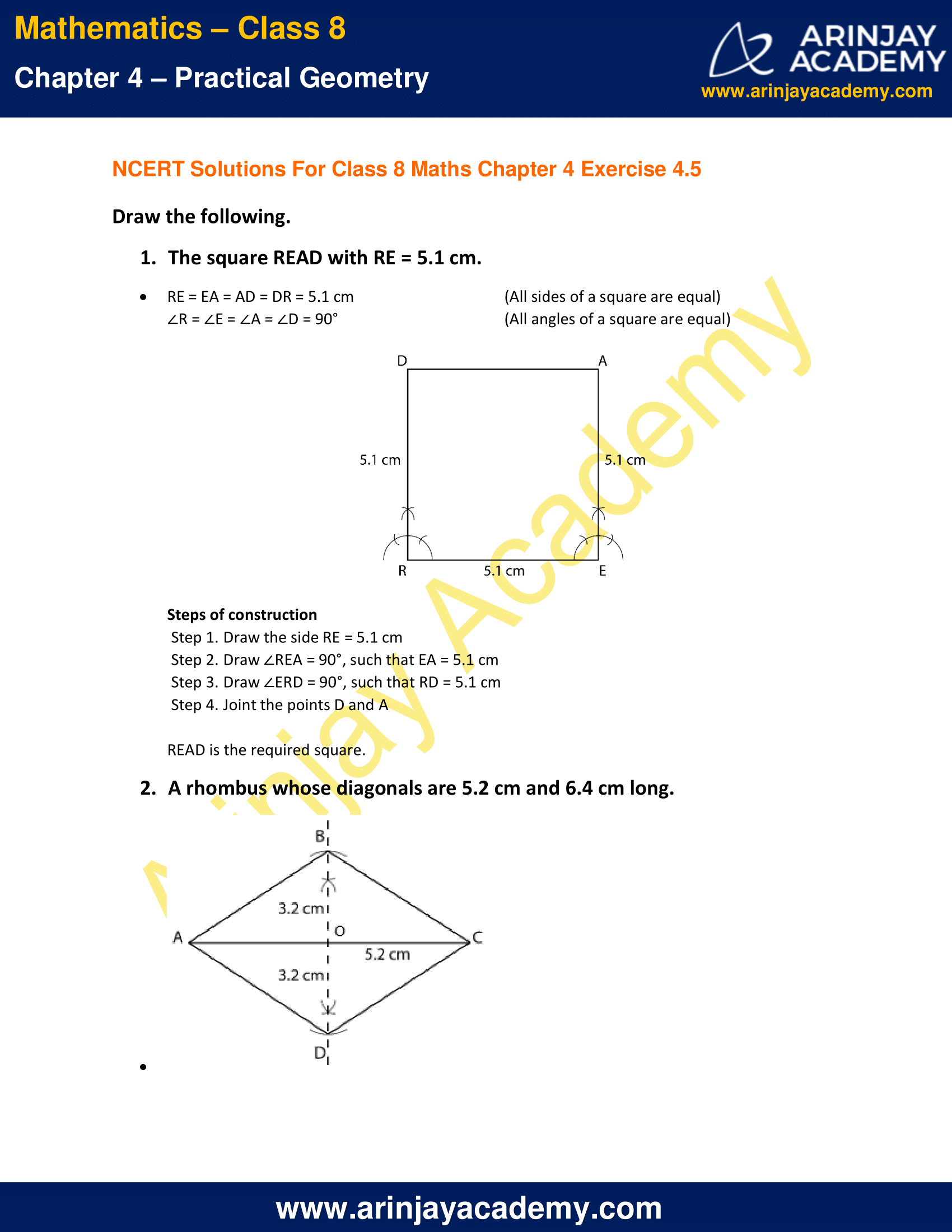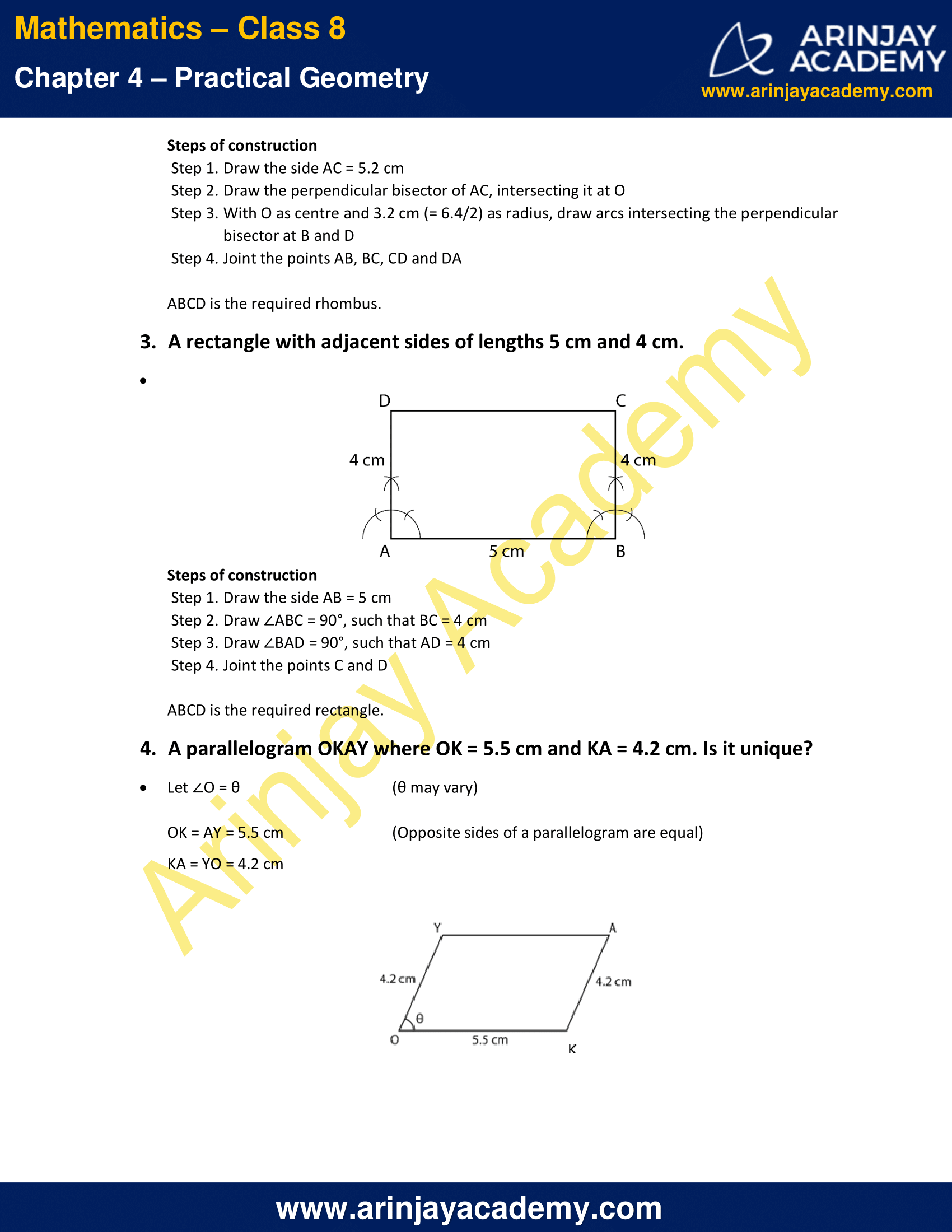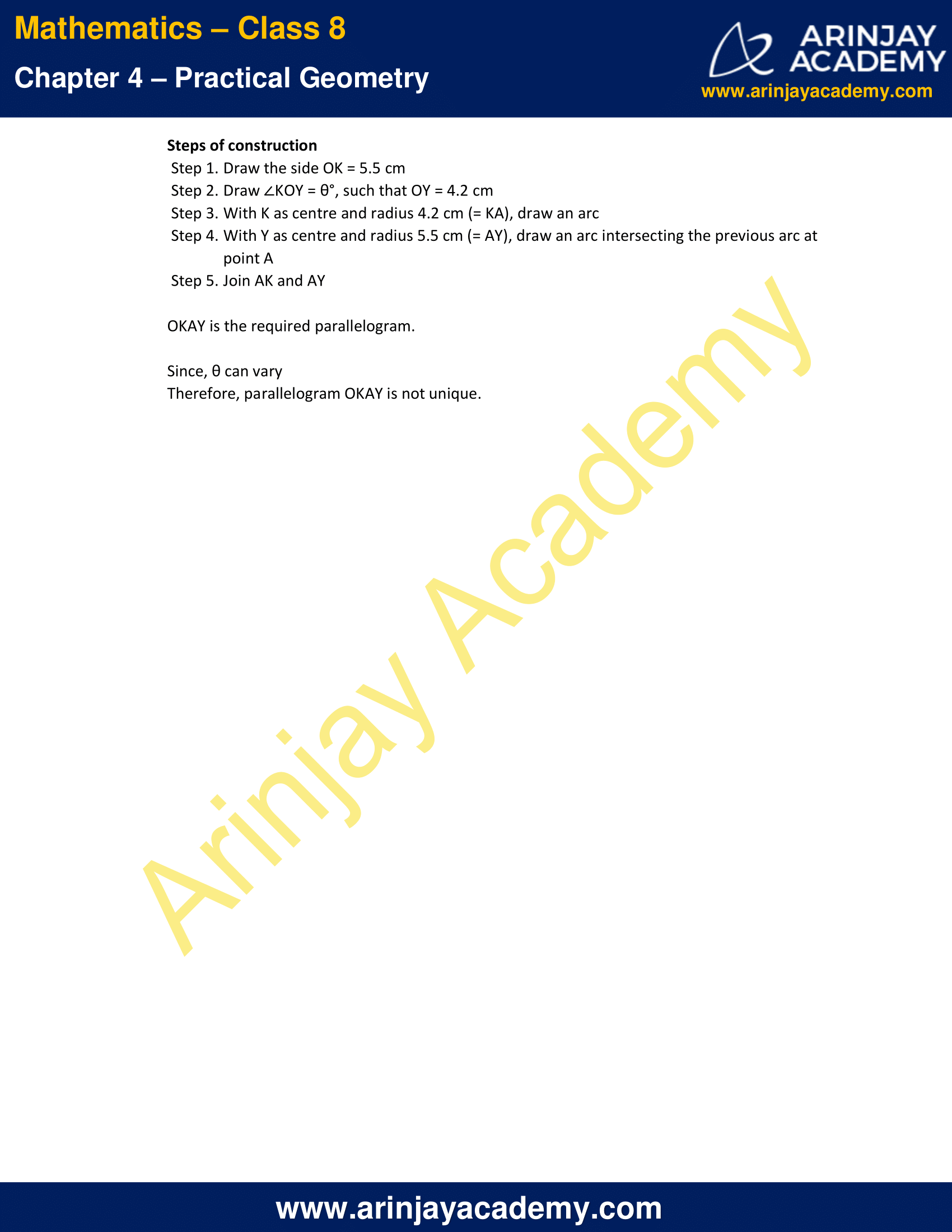NCERT Solutions for Class 8 Maths Chapter 4 Exercise 4.5 – Practical Geometry

Draw the following.

1. The square READ with RE = 5.1 cm.

Solution:

RE = EA = AD = DR = 5.1 cm (All sides of a square are equal)
∠R = ∠E = ∠A = ∠D = 90°  (All angles of a square are equal)Steps of construction

• Step 1. Draw the side RE = 5.1 cm
• Step 2. Draw ∠REA = 90°, such that EA = 5.1 cm
• Step 3. Draw ∠ERD = 90°, such that RD = 5.1 cm
• Step 4. Joint the points D and A

2. A rhombus whose diagonals are 5.2 cm and 6.4 cm long.

Solution: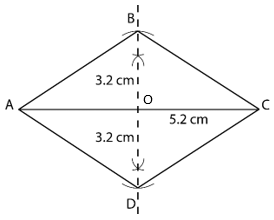Steps of construction

• Step 1. Draw the side AC = 5.2 cm
• Step 2. Draw the perpendicular bisector of AC, intersecting it at O
• Step 3. With O as centre and 3.2 cm (= 6.4/2) as radius, draw arcs intersecting the perpendicular bisector at B and D
• Step 4. Joint the points AB, BC, CD and DA

ABCD is the required rhombus.

3. A rectangle with adjacent sides of lengths 5 cm and 4 cm.

Solution:Steps of construction

• Step 1. Draw the side AB = 5 cm
• Step 2. Draw ∠ABC = 90°, such that BC = 4 cm
• Step 3. Draw ∠BAD = 90°, such that AD = 4 cm
• Step 4. Joint the points C and D

ABCD is the required rectangle.

4. A parallelogram OKAY where OK = 5.5 cm and KA = 4.2 cm. Is it unique?

Solution:

Let ∠O = θ (θ may vary)
OK = AY = 5.5 cm                               (Opposite sides of a parallelogram are equal)
KA = YO = 4.2 cm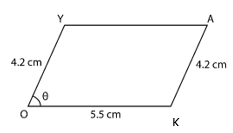Steps of construction

• Step 1. Draw the side OK = 5.5 cm
• Step 2. Draw ∠KOY = θ°, such that OY = 4.2 cm
• Step 3. With K as centre and radius 4.2 cm (= KA), draw an arc
• Step 4. With Y as centre and radius 5.5 cm (= AY), draw an arc intersecting the previous arc at point A
• Step 5. Join AK and AY

OKAY is the required parallelogram.
Since, θ can vary
Therefore, parallelogram OKAY is not unique.

The next Exercise for NCERT Solutions for Class 8 Maths Chapter 5 Exercise 5.1 – Data Handling can be accessed by clicking here

NCERT Solutions for Class 8 Maths Chapter 4 Exercise 4.5 – Practical Geometry